# Selina solutions for Concise Physics Class 8 ICSE chapter 5 - Light Energy [Latest edition]

#### Chapters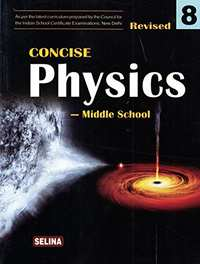## Chapter 5: Light Energy

Objective Questions [Pages 98 - 99]

### Selina solutions for Concise Physics Class 8 ICSE Chapter 5 Light Energy Objective Questions [Pages 98 - 99]

#### Write true or false

Objective Questions | Q 1.01 | Page 98

Water is optically denser than glass.

• True

• False

Objective Questions | Q 1.02 | Page 98

A ray of light when passes from glass to air, bends towards the normal.

• True

• False

Objective Questions | Q 1.03 | Page 98

The speed of light is more in glass than in water.

• True

• False

Objective Questions | Q 1.04 | Page 98

The depth of a pond when seen from above appears to be less.

• True

• False

Objective Questions | Q 1.05 | Page 98

Light travels at a lower speed in water than in air.

• True

• False

Objective Questions | Q 1.06 | Page 98

Light travels in the same straight line path while passing through different media.

• True

• False

Objective Questions | Q 1.07 | Page 98

At the point of incidence, a line drawn at right angles to the surface, separating the two media, is called the normal.

• True

• False

Objective Questions | Q 1.08 | Page 98

The angle formed between the normal and the refracted ray is known as the angle of incidence.

• True

• False

Objective Questions | Q 1.1 | Page 98

Image is formed by a mirror due to refraction of light.

• True

• False

Objective Questions | Q 1.11 | Page 98

Rays of light incident parallel to the principal axis pass through the focus after reflection from a concave mirror.

• True

• False

Objective Questions | Q 1.12 | Page 98

A convex mirror is used as a shaving mirror.

• True

• False

Objective Questions | Q 1.13 | Page 98

The focal length of a convex mirror is equal to its radius of curvature.

• True

• False

Objective Questions | Q 1.15 | Page 98

A concave mirror converges the light-rays, but a convex mirror diverges them.

• True

• False

Objective Questions | Q 1.16 | Page 98

A virtual image formed by a spherical mirror is always erect and situated behind the mirror.

• True

• False

Objective Questions | Q 2.01 | Page 98

Water is optically .......... than air.

Objective Questions | Q 2.02 | Page 98

Air is optically .......... than glass.

Objective Questions | Q 2.03 | Page 98

When a ray of light travels from water to air, it bends ............. the normal.

Objective Questions | Q 2.04 | Page 98

When a ray of light travels from air to glass, it bends .......... the normal.

Objective Questions | Q 2.05 | Page 98

When white light passes through a prism, it ............

Objective Questions | Q 2.06 | Page 98

The splitting of white light into its constituent colours is called .............

Objective Questions | Q 2.07 | Page 98

A .............. mirror is obtained on silvering the outer surface of a part of a hollow glass sphere.

Objective Questions | Q 2.08 | Page 98

Radius of curvature of a spherical mirror is ............. its focal length.

Objective Questions | Q 2.09 | Page 98

The angle of incidence for a ray of light passing through the centre of curvature of a spherical mirror is ...........

Objective Questions | Q 2.1 | Page 98

A ............ mirror always forms a virtual image.

Objective Questions | Q 2.11 | Page 98

A concave mirror forms a virtual image for an object placed ...............................

Objective Questions | Q 3.01 | Page 98

Match the Following

 Column A Column B (a) white Light (1) Convex mirror (b) Refraction (2) Concave mirror (c) Virtual images (3) refraction (d) Real images (4)  spectrum (e) Prism (5) ray of light from glass to air

#### Select the correct alternative

Objective Questions | Q 4.01 | Page 98

The speed of light in air or vacuum is

• 3 × 10M s-1

• 2.25 × 108 m s-1

• 332 ms-1

• 2.0 × 108 ms-1

Objective Questions | Q 4.02 | Page 99

A ray of light moving from an optically rarer to a denser medium

• bends away from the normal

• bends towards the normal

• remains undeviated

• none of the above

Objective Questions | Q 4.03 | Page 99

The angle between the normal and refracted ray is called

• angle of deviation

• angle of incidence

• angle of refraction

• the angle of emergence.

Objective Questions | Q 4.04 | Page 99

The property of splitting of white light into its seven constituent colours is known as

• rectilinear propagation

• refraction

• reflection

• dispersion

Objective Questions | Q 4.05 | Page 99

The seven colours in the spectrum of sunlight in order, are represented as :

• VIBGYOR

• VIGYBOR

• BIVGYOR

• RYOBIVG

Objective Questions | Q 4.06 | Page 99

A ray of light passing through centre of curvature of a spherical mirror, after reflection

• passes through the focus

• passes through the pole

• becomes parallel to the principal axis

• retraces its own path.

Objective Questions | Q 4.07 | Page 99

If the radius of curvature of a concave mirror is 20 cm, its focal length is:

• 10 cm

• 20 cm

• 40 cm

• 80 cm

Objective Questions | Q 4.08 | Page 99

The image formed by a convex mirror is

• erect and diminished

• erect and enlarged

• inverted and diminished

• inverted and enlarged.

Objective Questions | Q 4.09 | Page 99

The image formed by a concave mirror is of the same size as the object, if the object is placed

• at the focus

• between the pole and focus

• between the focus and centre of curvature

• at the centre of curvature.

Objective Questions | Q 4.1

A convex mirror is used

•  as a shaving mirror

•  as a head mirror by a dentist

• as a rear view mirror by a driver

•  as a reflector in torch

Short/long Answer Question [Pages 99 - 101]

### Selina solutions for Concise Physics Class 8 ICSE Chapter 5 Light Energy Short/long Answer Question [Pages 99 - 101]

Short/long Answer Question | Q 1 | Page 99

State the speed of light in (a) air, (b) water, and (c) glass

Short/long Answer Question | Q 2 | Page 99

How does the speed of light determine the optical density of a medium?

Short/long Answer Question | Q 3 | Page 99

Which is optically denser: water or air? Give reason

Short/long Answer Question | Q 4 | Page 99

Out of air and glass, which is optically rarer? Give reason.

Short/long Answer Question | Q 5 | Page 99

What do you understand by refraction of light?

Short/long Answer Question | Q 6 | Page 99

Describe an experiment to show that a light ray bends when it passes from one transparent medium into another transparent medium.

Short/long Answer Question | Q 7 | Page 99

Draw a ray diagram to show that the depth of a vessel containing water when seen from above, appears to be less than its real depth.

Short/long Answer Question | Q 8 | Page 99

Define the following terms :
Incident ray, Refracted ray, Angle of incidence, Angle of refraction.

Short/long Answer Question | Q 9 | Page 99

A ray of light falls normally on a glass slab. What is the angle of incidence?

Short/long Answer Question | Q 10 | Page 99

A ray of light travels from a rarer medium to a denser medium. How will it bend?

Short/long Answer Question | Q 11 | Page 100

A ray of light travels from a denser medium to a rarer medium. How will it bend?

Short/long Answer Question | Q 12 | Page 100

The diagram given below in fig shows a ray of light AO falling on a surface separating two media. Draw the refracted ray in each, case.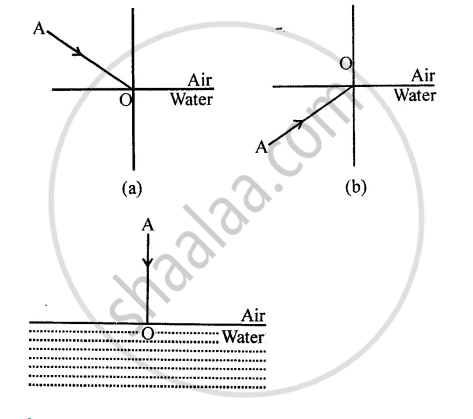Short/long Answer Question | Q 13 | Page 100

Draw a diagram showing the refraction of a light ray from water to glass. Label on it the incident ray, the angle of incidence (/), and the angle of refraction (r)

Short/long Answer Question | Q 14 | Page 100

The diagram in figure shows a ray of light AO falling on a rectangular glass slab PQRS. Complete the diagram till the ray of light emerges out of the slab. Label on the diagram the incident ray, the refracted ray and the emergent ray.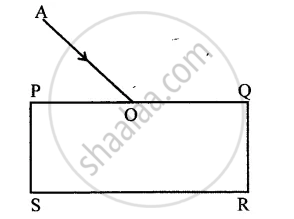#### Explain the following

Short/long Answer Question | Q 15.1 | Page 100

A coin placed at the bottom of a vessel appears to be raised when water is poured in the vessel.

Short/long Answer Question | Q 15.2 | Page 100

A straight stick partly dipped in water obliquely, appears to be bent at the surface of water.

Short/long Answer Question | Q 15.3 | Page 100

The sun is seen before the sunrise and after the sunset.

Short/long Answer Question | Q 16 | Page 100

What is mirage? Give a reason for its formation?

Short/long Answer Question | Q 17 | Page 100

What is a prism? Draw a ray diagram to show the refraction of a light ray through a prism.

Short/long Answer Question | Q 18 | Page 100

What do you mean by the term dispersion?

Short/long Answer Question | Q 19 | Page 100

A ray of white light falls on a prism. Draw a ray diagram to show that the prism disperses the white light.

Short/long Answer Question | Q 20 | Page 100

In figure AO is the ray of white light falling on a prism PQR. Complete the diagram till the light emerges out from the prism and falls on the screen.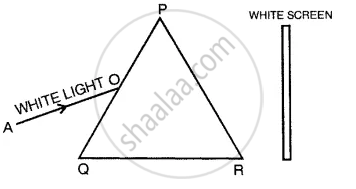Short/long Answer Question | Q 21 | Page 100

What do you understand by the term spectrum? Name the various colours present in the spectrum of sunlight.

Short/long Answer Question | Q 22 | Page 100

You are given a disc divided into seven sectors with colours violet, indigo, blue, green, yellow, orange and red in them. What would be its colour when it is rotated rapidly?

Short/long Answer Question | Q 23 | Page 100

State the two laws of reflection of light.

Short/long Answer Question | Q 24 | Page 100

What is a spherical mirror?

Short/long Answer Question | Q 25 | Page 100

State the two kinds of spherical mirror and distinguish them with the aid of proper diagrams.

Short/long Answer Question | Q 26 | Page 100

Explain the following terms :
Pole, Centre of curvature, Radius of curvature, Principal axis. Show them on separate diagrams for each of the concave and convex mirrors

Short/long Answer Question | Q 27 | Page 100

What do you understand by the focus and focal length of a spherical mirror? Show them on the separate diagrams for each of a concave mirror and a convex mirror.

Short/long Answer Question | Q 28 | Page 100

Draw suitable diagrams to illustrate how a beam of light incident parallel to the principal axis is reflected by:
(a) a concave mirror, and (b) a convex mirror

Short/long Answer Question | Q 29 | Page 100

How is a spherical mirror used to converge a beam of light at a point? Name the type of mirror used.

Short/long Answer Question | Q 30 | Page 101

How is a spherical mirror used to diverge a beam of light from a point? Name the type of mirror used.

Short/long Answer Question | Q 31 | Page 101

State the direction of incident ray which after reflection from a spherical mirror gets reflected along its own path. Give a reason.

Short/long Answer Question | Q 32 | Page 101

How is the focal length of a spherical mirror related to its radius

Short/long Answer Question | Q 33 | Page 101

The diagram (figure) given below shows two parallel rays 1 and 2 incident on (a) a concave mirror, (b) a convex mirror. Draw the reflected rays and mark the focus by the symbol F.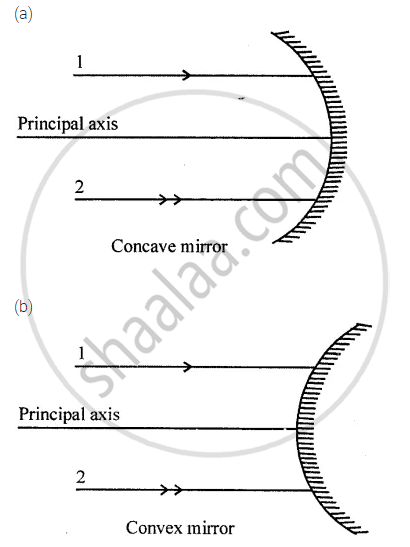Short/long Answer Question | Q 34 | Page 101

Complete the following diagrams in figure by drawing the reflected rays for the incident rays 1 and 2 if F is the focus and C is the centre of curvature.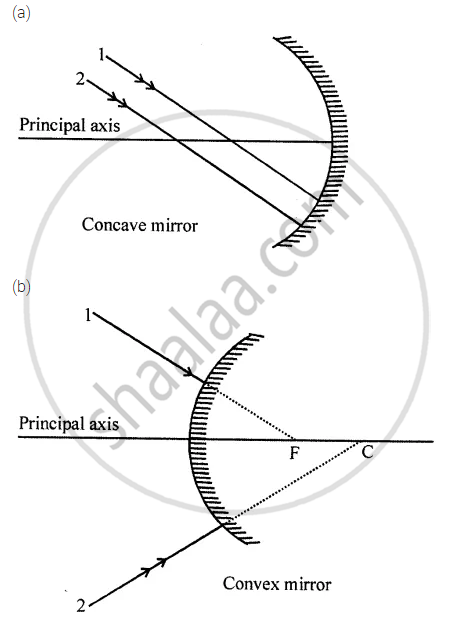Short/long Answer Question | Q 35 | Page 101

Which are the two convenient rays that are chosen to construct the image by a spherical mirror for a given object? Explain with the help of suitable ray diagrams.

Short/long Answer Question | Q 36 | Page 101

Draw a ray diagram to show the formation of the image of an object placed beyond the centre of curvature of a concave mirror. State the position, size and nature of the image.

Short/long Answer Question | Q 37 | Page 101

Draw a ray diagram to show the formation of image of an object placed at the centre of curvature of a concave mirror. State the position, size and nature of the image.

Short/long Answer Question | Q 38 | Page 101

Draw a ray diagram to show the formation of image of an object placed between the focus and centre of curvature of a concave mirror. State the position, size and nature of the image.

Short/long Answer Question | Q 39 | Page 101

Draw a ray diagram to show the formation of image of an object placed between the pole and focus of a concave mirror. State the position, size and nature of the image.

Short/long Answer Question | Q 40 | Page 101

Draw a ray diagram to show the formation of image of an object placed on the principal axis of a convex mirror. State the position, size and nature of the image. What happens to the image as the object is moved away from the mirror?

Short/long Answer Question | Q 41 | Page 101

Draw separate diagrams for the formation of virtual image of an object by convex mirror. State the difference between concave and convex image.

Short/long Answer Question | Q 42 | Page 101

Name the mirror which always forms an erect and virtual image. What is the size of the image as compared to that of the object?

Short/long Answer Question | Q 43 | Page 101

Name the mirror which forms an erect, virtual and enlarged image of an object. What is the position of object relative to the mirror?

Short/long Answer Question | Q 44 | Page 101

What is a real image? Name the mirror which can be used to obtain the real image of an object. What should be the position of the object relative to the mirror?

Short/long Answer Question | Q 45 | Page 101

How can a concave mirror be used to obtain a virtual image of an object? Draw a diagram to illustrate your answer.

Short/long Answer Question | Q 46 | Page 101

State two uses of a concave mirror.

Short/long Answer Question | Q 47 | Page 101

State two uses of a convex mirror.

Short/long Answer Question | Q 48 | Page 101

A driver uses a convex mirror as a rearview mirror. Explain the reason with the help of a ray diagram.

Short/long Answer Question | Q 49 | Page 101

State the kind of mirror used
(a) by a dentist, and
(b) as a street light reflector.

Short/long Answer Question | Q 50 | Page 101

Name the kind of mirror used to obtain
(a) a real and enlarged image
(b) a virtual and enlarged image
(c) a real and diminished image, and
(d) a virtual and diminished image.

## Chapter 5: Light Energy## Selina solutions for Concise Physics Class 8 ICSE chapter 5 - Light Energy

Selina solutions for Concise Physics Class 8 ICSE chapter 5 (Light Energy) include all questions with solution and detail explanation. This will clear students doubts about any question and improve application skills while preparing for board exams. The detailed, step-by-step solutions will help you understand the concepts better and clear your confusions, if any. Shaalaa.com has the CISCE Concise Physics Class 8 ICSE solutions in a manner that help students grasp basic concepts better and faster.

Further, we at Shaalaa.com provide such solutions so that students can prepare for written exams. Selina textbook solutions can be a core help for self-study and acts as a perfect self-help guidance for students.

Concepts covered in Concise Physics Class 8 ICSE chapter 5 Light Energy are Refraction of Light, Dispersion of Light Through Prism and Formation of Spectrum, Spherical Mirrors, Some Terms Related to Refraction of Light, Atmospheric Refraction, Application of Atmospheric Refraction, Refraction of Light Through a Rectangular Glass Slab, Refraction of Light Through a Prism, Effects of Refraction, Rules for the Construction of Image Formed by a Spherical Mirror, Image Formation by Convex Mirror, Law of Refraction of Light, Image Formation by Concave Mirror, Law of Refraction of Light, Speed of Light, Concept of Prism, Dispersion of Light and Its Causes, Formation of Image by Reflection: Real and Virtual Image, Convex Mirror, Concave Mirror.

Using Selina Class 8 solutions Light Energy exercise by students are an easy way to prepare for the exams, as they involve solutions arranged chapter-wise also page wise. The questions involved in Selina Solutions are important questions that can be asked in the final exam. Maximum students of CISCE Class 8 prefer Selina Textbook Solutions to score more in exam.

Get the free view of chapter 5 Light Energy Class 8 extra questions for Concise Physics Class 8 ICSE and can use Shaalaa.com to keep it handy for your exam preparation